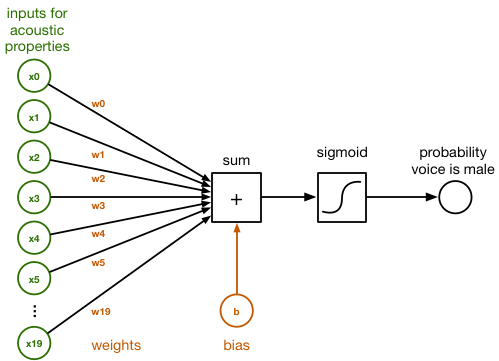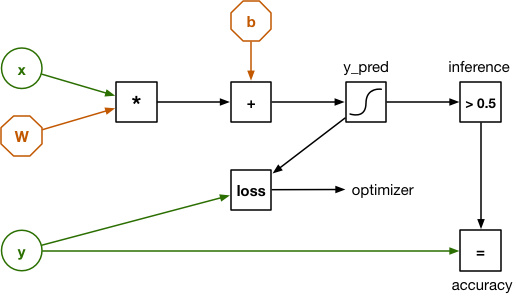# 在iOS平台上使用TensorFlow

### 2017 - 04 - 17

Posted by Matthijs Hollemans## TensorFlow是什么，我们为何需要加以使用？

TensorFlow是一套用于构建计算性图形，从而实现机器学习的软件资源库。

## 利用逻辑回归实现二元分类``````sum = x*w + x*w + x*w + ... + x*w + b
``````

``````y_pred = 1 / (1 + exp(-sum))
``````## Tensorflow到底是什么？

Tensor一词本义为张量，而此计算图中全部数据流皆以张量形式存在。所谓张量，其实际代表的就是一个n维数组。我曾经提到W是一项权重矩阵，但从TensorFlow的角度来看，其实际上属于一项二阶张量——换言之，一个二组数组。

• 一个标量代表一个零阶张量。

• 一个向量代表一个一阶张量。

• 一个矩阵代表一个二阶张量。

• 一个三维数组代表一个三阶张量。

## 安装TensorFlow

``````brew install python3
``````

Python也拥有自己的软件包管理器，即pip，我们将利用它安装我们所需要的其它软件包。在终端中输入以下命令：

``````pip3 install numpy
pip3 install scipy
pip3 install scikit-learn
pip3 install pandas
pip3 install tensorflow
``````

NumPy是一套用于同n级数组协作的库。听起来耳熟吗？NumPy并非将其称为张量，但之前提到了数组本身就是一种张量。TensorFlow Python API就建立在NumPy基础之上。

SciPy是一套用于数值计算的库。其它一些软件包的起效需要以之为基础。

pandas负责数据集的加载与清理工作。

scikit-learn在某种意义上可以算作TensorFlow的竞争对手，因为其同样是一套用于机器学习的库。我们之所以在本项目中加以使用，是因为它具备多项便利的功能。由于TensorFlow与scikit-learn皆使用NumPy数组，因为二者能够顺畅实现协作。

``````import tensorflow as tf
a = tf.constant([1, 2, 3])
b = tf.constant([4, 5, 6])
sess = tf.Session(config=tf.ConfigProto(log_device_placement=True))
print(sess.run(a + b))
``````

``````python3 tryit.py
``````

``````[5 7 9]
``````

``````W tensorflow/core/platform/cpu_feature_guard.cc:45] The TensorFlow library  wasn't compiled to use SSE4.1 instructions, but these are available on your  machine and could speed up CPU computations.
``````• 以kHz为单位的平均频率

• 频率的标准差

• 频谱平坦度

• 频谱熵

• 峰度

• 声学信号中测得的最大基频

• 调制指数

• 等等……

## 建立一套训练集与测试集

• 向其交付来自数据集的全部示例。

• 衡量预测结果的错误程度。

• 根据loss调整权重。

``````import numpy as np               # 1
labels = (df["label"] == "male").values * 1    # 3
labels = labels.reshape(-1, 1)                 # 4
del df["label"]                  # 5
data = df.values

# 6
from sklearn.model_selection import train_test_split X_train,
X_test, y_train, y_test = train_test_split(data, labels,                                          test_size=0.3, random_state=123456)
np.save("X_train.npy", X_train)  # 7
np.save("X_test.npy", X_test)
np.save("y_train.npy", y_train)
np.save("y_test.npy", y_test)
``````

• 首先导入NumPy与pandas软件包。Pandas能够轻松实现CSV文件的加载，并对数据进行预处理。

• 利用pandas从voice.csv加载数据集并将其作为dataframe。此对象在很大程度上类似于电子表格或者SQL表。

• 这里的label列包含有该数据集的各项标签：即该示例为男声或者女声。在这里，我们将这些标签提取进一个新的NumPy数组当中。各原始标签为文本形式，但我们将其转化为数字形式，其中1=男声，0=女声。（这里的数字赋值方式可任意选择，在二元分类器中，我们通常使用1表示‘正’类，或者说我们试图检测的类。）

• 这里创建的新labels数组是一套一维数组，但我们的TensorFlow脚本则需要一套二维张量，其中3168行中每一行皆对应一列。因此我们需要在这里对数组进行“重塑”，旨在将其转化为二维形式。这不会对内存中的数据产生影响，而仅变化NumPy对数据的解释方式。

• 在完成label列之后，我们将其从dataframe当中移除，这样我们就只剩下20项用于描述输入内容的特征。我们还将把该dataframe转换为一套常规NumPy数组。

• 这里，我们利用来自scikit-learn的一项helper函数将data与labels数组拆分为两个部分。这种对数据集内各示例进行随机洗牌的操作基于random_state，即一类随机生成器。无论具体内容为何，但只要青筋相同内容，我们即创造出了一项可重复进行的实验。

• 最后，将四项新的数组保存为NumPy的二进制文件格式。现在我们已经拥有了一套训练集与一套测试集！

``````python3 split_data.py
``````

## 建立计算图

``````import numpy as np
import tensorflow as tf
``````

``````num_inputs = 20
num_classes = 1

with tf.name_scope("inputs"):
x = tf.placeholder(tf.float32, [None, num_inputs], name="x-input")
y = tf.placeholder(tf.float32, [None, num_classes], name="y-input")
``````

``````with tf.name_scope("model"):
W = tf.Variable(tf.zeros([num_inputs, num_classes]), name="W")
b = tf.Variable(tf.zeros([num_classes]), name="b")
``````

``````y_pred = tf.sigmoid(tf.matmul(x, W) + b)
``````

``````with tf.name_scope("loss-function"):
loss = tf.losses.log_loss(labels=y, predictions=y_pred)
loss += regularization * tf.nn.l2_loss(W)
``````

``````with tf.name_scope("hyperparameters"):
regularization = tf.placeholder(tf.float32, name="regularization")
learning_rate = tf.placeholder(tf.float32, name="learning-rate")
``````

Hyperparameters允许大家对这套模型及其具体训练方式进行配置。其之所以被称为“超”参数，是因为与常见的W与b参数不同，其并非由模型自身所学习——大家需要自行将其设置为适当的值。

``````with tf.name_scope("train"):
train_op = optimizer.minimize(loss)
``````

``````with tf.name_scope("score"):
correct_prediction = tf.equal(tf.to_float(y_pred > 0.5), y)
accuracy = tf.reduce_mean(tf.to_float(correct_prediction), name="accuracy")
``````

``````with tf.name_scope("inference"):
inference = tf.to_float(y_pred > 0.5, name="inference")
``````

## 训练分类器

``````with tf.Session() as sess:
tf.train.write_graph(sess.graph_def, checkpoint_dir, "graph.pb", False)

sess.run(init)

step = 0
while True:
# here comes the training code
``````

``````perm = np.arange(len(X_train))
np.random.shuffle(perm)
X_train = X_train[perm]
y_train = y_train[perm]
``````

``````        feed = {x: X_train, y: y_train, learning_rate: 1e-2,
regularization: 1e-5}
sess.run(train_op, feed_dict=feed)
``````

``````   if step % print_every == 0:
train_accuracy, loss_value = sess.run([accuracy, loss],                                         feed_dict=feed)
print("step: %4d, loss: %.4f, training accuracy: %.4f" % \
(step, loss_value, train_accuracy))
``````

``````if step % save_every == 0:
checkpoint_file = os.path.join(checkpoint_dir, "model")
saver.save(sess, checkpoint_file)
print("*** SAVED MODEL ***")
``````

``````python3 train.py
``````

``````Training set size: (2217, 20)
Initial loss: 0.693146
step:    0, loss: 0.7432, training accuracy: 0.4754
step: 1000, loss: 0.4160, training accuracy: 0.8904
step: 2000, loss: 0.3259, training accuracy: 0.9170
step: 3000, loss: 0.2750, training accuracy: 0.9229
step: 4000, loss: 0.2408, training accuracy: 0.9337
step: 5000, loss: 0.2152, training accuracy: 0.9405
step: 6000, loss: 0.1957, training accuracy: 0.9553
step: 7000, loss: 0.1819, training accuracy: 0.9594
step: 8000, loss: 0.1717, training accuracy: 0.9635
step: 9000, loss: 0.1652, training accuracy: 0.9666
*** SAVED MODEL ***
step: 10000, loss: 0.1611, training accuracy: 0.9702
step: 11000, loss: 0.1589, training accuracy: 0.9707
. . .
``````

## 实际效果如何？

``````import numpy as np
import tensorflow as tf from sklearn
import metrics
``````

``````with tf.Session() as sess:
graph_file = os.path.join(checkpoint_dir, "graph.pb")
with tf.gfile.FastGFile(graph_file, "rb") as f:
graph_def = tf.GraphDef()
tf.import_graph_def(graph_def, name="")
``````

TensorFlow可能会将其数据保存为协议缓冲文件（扩展名为.pb），因此我们可以使用部分helper代码以加载此文件并将其作为计算图导入至会话当中。

``````W = sess.graph.get_tensor_by_name("model/W:0")
b = sess.graph.get_tensor_by_name("model/b:0")

checkpoint_file = os.path.join(checkpoint_dir, "model")
saver = tf.train.Saver([W, b])
saver.restore(sess, checkpoint_file)
``````

``````x = sess.graph.get_tensor_by_name("inputs/x-input:0")
y = sess.graph.get_tensor_by_name("inputs/y-input:0")
accuracy = sess.graph.get_tensor_by_name("score/accuracy:0")
inference = sess.graph.get_tensor_by_name("inference/inference:0")
``````

``````feed = {x: X_test, y: y_test}
print("Test set accuracy:", sess.run(accuracy, feed_dict=feed))
``````

``````predictions = sess.run(inference, feed_dict={x: X_test})
print("Classification report:")
print(metrics.classification_report(y_test.ravel(), predictions))
print("Confusion matrix:")
print(metrics.confusion_matrix(y_test.ravel(), predictions))
``````

``````\$ python3 test.py
Test set accuracy: 0.958991
Classification report:
precision    recall  f1-score   support
0       0.98      0.94      0.96       474
1       0.94      0.98      0.96       477
avg / total       0.96      0.96      0.96       951
Confusion matrix:
[[446  28]
[ 11 466]]
``````

## 在iOS上使用TensorFlow

``````sudo xcode-select -s /Applications/Xcode.app/Contents/Developer
``````

TensorFlow利用一款名为bazel的工具进行构建，bazel则要求配合Java JDK 8。大家可以利用Homebrew轻松安装必要的软件包：

``````brew cask install java brew install bazel brew install automake brew install libtool
``````

``````cd /Users/matthijs git clone https://github.com/tensorflow/tensorflow -b r1.0
``````

``````cd tensorflow ./configure
``````

``````Please specify the location of python. [Default is /usr/bin/python]:
``````

``````Please specify optimization flags to use during compilation [Default is  -march=native]:
``````

## 构建静态库

``````tensorflow/contrib/makefile/build_all_ios.sh
``````

``````bazel build tensorflow/python/tools:freeze_graph bazel build tensorflow/python/tools:optimize_for_inference
``````

``````bazel build --copt=-march=native -c opt //tensorflow/tools/pip_package:build_pip_package  bazel-bin/tensorflow/tools/pip_package/build_pip_package /tmp/tensorflow_pkg
``````

``````pip3 uninstall tensorflow sudo -H pip3 install /tmp/tensorflow_pkg/tensorflow-1.0.0-XXXXXX.whl
``````

## “冻结”计算图

``````bazel-bin/tensorflow/python/tools/freeze_graph \
--input_graph=/tmp/voice/graph.pb --input_checkpoint=/tmp/voice/model \
--output_node_names=model/y_pred,inference/inference --input_binary \
--output_graph=/tmp/voice/frozen.pb
``````

``````bazel-bin/tensorflow/python/tools/optimize_for_inference \
--input=/tmp/voice/frozen.pb --output=/tmp/voice/inference.pb \
--input_names=inputs/x --output_names=model/y_pred,inference/inference \
--frozen_graph=True
``````

## iOS应用

1. 此应用利用Objective-C++编写（其源文件扩展名为.mm）。在编写时尚不存在面向TensorFlow的Swift API，因此只能使用C++。

2. 其中的inference.pb文件已经包含在项目当中。如果需要，大家也可以直接将自有版本的inference.pb复制到此项目的文件夹之内。

3. 此应用与Accelerate.framework相链接。

4. 此应用与我们此前已经编译完成的几套静态库相链接。

``````/Users/matthijs/tensorflow/tensorflow/contrib/makefile/gen/protobuf_ios/lib/ libprotobuf-lite.a   /Users/matthijs/tensorflow/tensorflow/contrib/makefile/gen/protobuf_ios/lib/ libprotobuf.a
``````

``````~/tensorflow ~/tensorflow/tensorflow/contrib/makefile/downloads  ~/tensorflow/tensorflow/contrib/makefile/downloads/eigen  ~/tensorflow/tensorflow/contrib/makefile/downloads/protobuf/src  ~/tensorflow/tensorflow/contrib/makefile/gen/proto
``````

• Enable Bitcode: No

• Warnings / Documentation Comments: No

• Warnings / Deprecated Functions: No

Bitcode目前尚不受TensorFLow的支持，所以我决定将其禁用。我还关闭了警告选项，否则在编译应用时会出现一大票问题提示。（禁用之后，大家仍然会遇到几项关于值转换问题的警告。大家当然也可以将其一并禁用，但我个人还是希望多少了解一点其中的错误。）

## 使用 TensorFlow C++ API

TensorFlow for iOS由C++编写而成，但其中需要编写的C++代码量其实——幸运的是——并不多。一般来讲，大家只需要完成以下工作：

1. 从.pb文件中加载计算图与权重值。

2. 利用此计算图创建一项会话。

3. 将您的数据放置在一个输入张量内。

4. 在一个或者多个节点上运行计算图。

5. 从输出结果张量中获取结果。

``````- (BOOL)loadGraphFromPath:(NSString *)path
{
auto status = ReadBinaryProto(tensorflow::Env::Default(),                                    path.fileSystemRepresentation, &graph);
if (!status.ok()) {
return NO;
}
return YES;
}
``````

``````Error adding graph to session: No OpKernel was registered to support Op  'L2Loss' with these attrs.  Registered devices: [CPU], Registered kernels:   <no registered kernels>
``````

``````- (BOOL)createSession
{
tensorflow::SessionOptions options;
auto status = tensorflow::NewSession(options, &session);
if (!status.ok()) {
NSLog(@"Error creating session: %s",
status.error_message().c_str());
return NO;
}

status = session->Create(graph);
if (!status.ok()) {
NSLog(@"Error adding graph to session: %s",
status.error_message().c_str());
return NO;
}
return YES;
}
``````

``````- (void)predict:(float *)example {
tensorflow::Tensor x(tensorflow::DT_FLOAT,
tensorflow::TensorShape({ 1, 20 }));

auto input = x.tensor<float, 2>();
for (int i = 0; i < 20; ++i) {
input(0, i) = example[i];
}
``````

`````` std::vector<std::pair<std::string, tensorflow::Tensor>> inputs = {
{"inputs/x-input", x}
};

std::vector<std::string> nodes = {
{"model/y_pred"},
{"inference/inference"}
};

std::vector<tensorflow::Tensor> outputs;

auto status = session->Run(inputs, nodes, {}, &outputs);
if (!status.ok()) {
NSLog(@"Error running model: %s", status.error_message().c_str());
return;
}
``````

``````    pred, inf = sess.run([y_pred, inference], feed_dict={x: example})
``````

`````` auto y_pred = outputs.tensor<float, 2>();
NSLog(@"Probability spoken by a male: %f%%", y_pred(0, 0));

auto isMale = outputs.tensor<float, 2>();
if (isMale(0, 0)) {
NSLog(@"Prediction: male");
} else {
NSLog(@"Prediction: female");
}
}
``````

## 运行iOS应用

``````Node count: 9
Node 0: Placeholder 'inputs/x-input'
Node 1: Const 'model/W'
Node 2: Const 'model/b'
Node 3: MatMul 'model/MatMul'
Node 5: Sigmoid 'model/y_pred'
Node 6: Const 'inference/Greater/y'
Node 7: Greater 'inference/Greater'
Node 8: Cast 'inference/inference'
``````

``````Probability spoken by a male: 0.970405% Prediction: male  Probability spoken by a male: 0.005632% Prediction: female
``````

## iOS平台上TensorFlow的优势与缺点

TensorFlow是一款出色的机器学习模型训练工具，特别是对于那些不畏数学计算并乐于创建新型算法的朋友。要对规模更大的模型进行训练，大家甚至可以在云环境下使用TensorFLow。

1. 使用一款工具即可实现全部预期。大家可以同时利用TensorFlow训练模型并将其引用于设备之上。我们不再需要将自己的计算图移植至BNNS或者Metal等其它API处。在另一方面，大家则必须至少将部分Python代码“移植”为C++形式。

2. TensorFlow拥有众多超越BNNS或Metal的出色功能特性。

3. 大家可以在模拟器上对其进行测试。（Metal要求用户始终利用实机进行测试。）

1. 目前其尚不支持GPU。TensorFlow确实能够利用Acclerate框架以发挥CPU的向量指令优势，但在原始处理速度上仍无法与Metal相提并论。

2. TensorFLow API为C++，因此大家需要使用Objective-C++自行编写代码。

3. 大家无法直接利用Swift使用TensorFLow。C++ API相较于Python API存在更多局限性。这意味着大家无法在设备之上进行数据训练，因为反向传播所需要的自动梯度计算尚不受设备支持。但这并不是什么大问题，毕竟移动设备的硬件本身就不适合进行大规模数据集训练。

4. TensorFlow静态库的加入会令应用体积增加约40 MB。大家可以通过减少受支持操作的数量对其进行瘦身，但具体过程相当麻烦。另外这还不包含您模型本体的体积，这可能会让应用尺寸进一步膨胀。

## 在GPU上运行：使用Metal

``````W.eval().tofile("W.bin")     b.eval().tofile("b.bin")
``````

W.eval()负责计算W的当前值并将其返回为一个NumPy数组（过程与执行sess.run(W)完全一致）。此后，我们使用tofile()将该NumPy数据保存为一个二进制文件。好了，就是这么简单：-）

``````y_pred = sigmoid((W * x) + b)
``````

``````let W_url = Bundle.main.url(forResource: "W", withExtension: "bin"
let b_url = Bundle.main.url(forResource: "b", withExtension: "bin"
let W_data = try! Data(contentsOf: W_url!)
let b_data = try! Data(contentsOf: b_url!)
``````

``````let sigmoid = MPSCNNNeuronSigmoid(device: device)
let layerDesc = MPSCNNConvolutionDescriptor(
kernelWidth: 1, kernelHeight: 1,
inputFeatureChannels: 20, outputFeatureChannels: 1,
neuronFilter: sigmoid)

W_data.withUnsafeBytes { W in
b_data.withUnsafeBytes { b in
layer = MPSCNNFullyConnected(device: device,
convolutionDescriptor: layerDesc,
kernelWeights: W, biasTerms: b, flags: .none)
}
}
``````

``````let inputImgDesc = MPSImageDescriptor(channelFormat: .float16,
width: 1, height: 1, featureChannels: 20)let outputImgDesc = MPSImageDescriptor(channelFormat: .float16,
width: 1, height: 1, featureChannels: 1)

inputImage = MPSImage(device: device, imageDescriptor: inputImgDesc)
outputImage = MPSImage(device: device, imageDescriptor: outputImgDesc)
``````

``````func predict(example: [Float]) {
convert(example: example, to: inputImage)

let commandBuffer = commandQueue.makeCommandBuffer()
layer.encode(commandBuffer: commandBuffer, sourceImage: inputImage,
destinationImage: outputImage)
commandBuffer.commit()
commandBuffer.waitUntilCompleted()

let y_pred = outputImage.toFloatArray()
print("Probability spoken by a male: \(y_pred)%")

if y_pred > 0.5 {
print("Prediction: male")
} else {
print("Prediction: female")
}
}
``````

``````Probability spoken by a male: 0.970215% Prediction: male  Probability spoken by a male: 0.00568771% Prediction: female
``````

• 《在iOS上使用Deep MNIST与TensorFlow：上手指南》，由 Matt Rajca撰写。

• 《利用Metal Performance Shaders实现TensorFlow加速》，同样来自 Matt Rajca。《Tensorflow Cocoa示例》，由Aaron Hillegass撰写。

• TensorFlow资源库中的《TensorFlow iOS示例》。